﻿ Java Inheritance - Vehicle class hierarchy

# Java Inheritance Programming - Vehicle class hierarchy

## Java Inheritance: Exercise-9 with Solution

Write a Java program to create a vehicle class hierarchy. The base class should be Vehicle, with subclasses Truck, Car and Motorcycle. Each subclass should have properties such as make, model, year, and fuel type. Implement methods for calculating fuel efficiency, distance traveled, and maximum speed.

Sample Solution:

Java Code:

``````// Vehicle.java
// Parent class Vehicle

public abstract class Vehicle {
private String make;
private String model;
private int year;
private String fuelType;
private double fuelEfficiency;

public Vehicle(String make, String model, int year, String fuelType, double fuelEfficiency) {
this.make = make;
this.model = model;
this.year = year;
this.fuelType = fuelType;
this.fuelEfficiency = fuelEfficiency;
}

public String getMake() {
return make;
}

public String getModel() {
return model;
}

public int getYear() {
return year;
}

public String getFuelType() {
return fuelType;
}

public double getFuelEfficiency() {
return fuelEfficiency;
}

public abstract double calculateFuelEfficiency();

public abstract double calculateDistanceTraveled();

public abstract double getMaxSpeed();
}
```
```

Explanation:

This is an abstract class that serves as the parent class for the other vehicle classes. It contains five private instance variables (make, model, year, fuelType, and fuelEfficiency) and six public methods (a constructor, five getters for the instance variables, and three abstract methods). The abstract methods are meant to be overridden by child classes with specific implementations.

``````// Truck.java
// Child class Truck
public class Truck extends Vehicle {
private double cargoCapacity;

public Truck(String make, String model, int year, String fuelType, double fuelEfficiency, double cargoCapacity) {
super(make, model, year, fuelType, fuelEfficiency);
//Truck("Ford", "F-150", 2020, "GASOLINE", 8.112);
this.cargoCapacity = cargoCapacity;
}

public double getCargoCapacity() {
return cargoCapacity;
}

@Override
public double calculateFuelEfficiency() {
// implementation for fuel efficiency calculation for trucks
return getFuelEfficiency()*(1.0 / (1.0 + (getCargoCapacity() / 1000.0)));
}

@Override
public double calculateDistanceTraveled() {
// implementation for distance traveled calculation for trucks
return calculateFuelEfficiency() * getFuelEfficiency();
}

@Override
public double getMaxSpeed() {
// implementation for maximum speed calculation for trucks
return 80.0;
}
}
``````

Explanation:

The above class is a child class of Vehicle and extends the Vehicle class. It has an additional instance variable, cargoCapacity. The class has a constructor that accepts all the necessary parameters including cargo capacity. The class overrides the three abstract methods of the parent class and provides specific implementations of the methods.

``````// Car.java
// Child class Car

public class Car extends Vehicle {
private int numSeats;

public Car(String make, String model, int year, String fuelType, double fuelEfficiency, int numSeats) {
super(make, model, year, fuelType, fuelEfficiency);
this.numSeats = numSeats;
}
public int getNumSeats() {
return numSeats;
}
@Override
public double calculateFuelEfficiency() {
// implementation for fuel efficiency calculation for cars
return getFuelEfficiency() * (1.0 / (1.0 + (getNumSeats() / 5.0)));
}
@Override
public double calculateDistanceTraveled() {
// implementation for distance traveled calculation for cars
return calculateFuelEfficiency() * getFuelEfficiency();
}

@Override
public double getMaxSpeed() {
// implementation for maximum speed calculation for cars
return 120.0;
}
}
``````

Explanation:

The above class is another child class of Vehicle and extends the Vehicle class. It has an additional instance variable, numSeats. The class has a constructor that accepts all the necessary parameters including the number of seats. The class overrides the three abstract methods of the parent class and provides specific implementations of the methods.

``````// Motorcycle.java
// Child class Motorcycle

public class Motorcycle extends Vehicle {
private double engineDisplacement;

public Motorcycle(String make, String model, int year, String fuelType, double fuelEfficiency) {
super(make, model, year, fuelType, fuelEfficiency);
//  this.engineDisplacement = engineDisplacement;
}

public double getEngineDisplacement() {
return engineDisplacement;
}

@Override
public double calculateFuelEfficiency() {
// implementation for fuel efficiency calculation for motorcycles
return getFuelEfficiency() * (1.0 / (1.0 + (getEngineDisplacement() / 1000.0)));
}

@Override
public double calculateDistanceTraveled() {
// implementation for distance traveled calculation for cars
return calculateFuelEfficiency() * getFuelEfficiency();
}

@Override
public double getMaxSpeed() {
// implementation for maximum speed calculation for cars
return 80.0;
}
}
``````

Explanation:

This is also a child class of Vehicle and extends the Vehicle class. It has an additional instance variable, engineDisplacement. The class has a constructor that accepts all the necessary parameters. The class overrides the three abstract methods of the parent class and provides specific implementations of the methods.

``````// Main.java
// Main class
public class Main {
public static void main(String[] args) {

// Create instances of each vehicle type
Truck truck = new Truck("Tatra", "Tatra 810 4x4", 2020, "GASOLINE", 8.112, 4.5);
Car car = new Car("Volkswagen", "Virtus", 2019, "HYBRID", 6.123, 8);
Motorcycle motorcycle = new Motorcycle("Massimo Motor", "Warrior200", 2018, "GASOLINE", 2.1);

// Print the vehicle details and calculations
System.out.println("Truck Model: " + truck.getModel());
System.out.println("Fuel Efficiency: " + truck.calculateFuelEfficiency() + " mpg");
System.out.println("Distance Traveled: " + truck.calculateDistanceTraveled() + " miles");
System.out.println("Max Speed: " + truck.getMaxSpeed() + " mph\n");

System.out.println("Car Model: " + car.getModel());
System.out.println("Fuel Efficiency: " + car.calculateFuelEfficiency() + " mpg");
System.out.println("Distance Traveled: " + car.calculateDistanceTraveled() + " miles");
System.out.println("Max Speed: " + car.getMaxSpeed() + " mph\n");

System.out.println("Motorcycle Model: " + motorcycle.getModel());
System.out.println("Fuel Efficiency: " + motorcycle.calculateFuelEfficiency() + " mpg");
System.out.println("Distance Traveled: " + motorcycle.calculateDistanceTraveled() + " miles");
System.out.println("Max Speed: " + motorcycle.getMaxSpeed() + " mph");
}
}
``````

Explanation:

The above class is the main class that contains the main method. It creates instances of each vehicle type, sets their values, and then prints their respective details and calculations such as fuel efficiency, distance traveled, and max speed.

Sample Output:

```Truck Model: Tatra 810 4x4
Fuel Efficiency: 8.075659532105526 mpg
Distance Traveled: 65.50975012444003 miles
Max Speed: 80.0 mph

Car Model: Virtus
Fuel Efficiency: 2.355 mpg
Distance Traveled: 14.419665 miles
Max Speed: 120.0 mph

Motorcycle Model: Warrior200
Fuel Efficiency: 2.1 mpg
Distance Traveled: 4.41 miles
Max Speed: 80.0 mph
```

Flowchart of Vehicle class: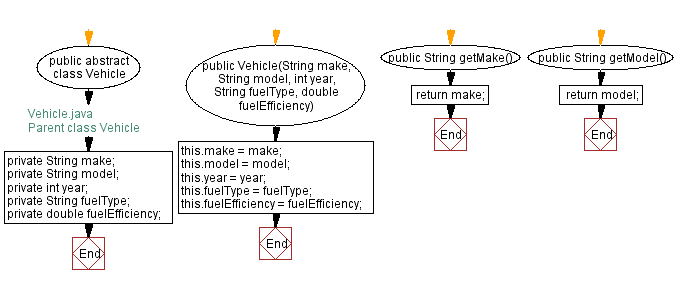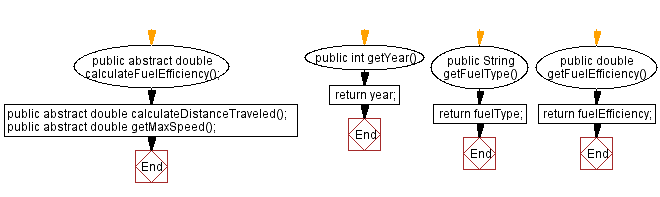Flowchart of Child class Truck: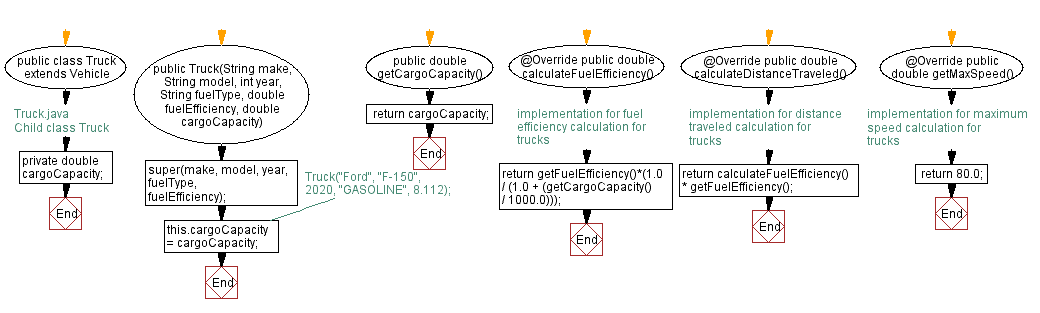Flowchart of Child class Car: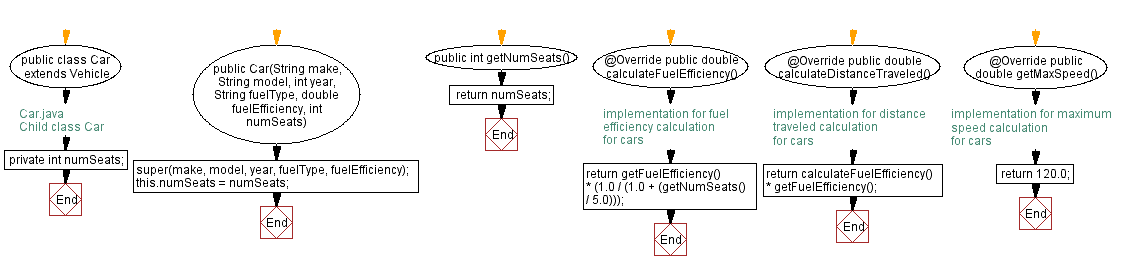Flowchart of Child class Motorcycle: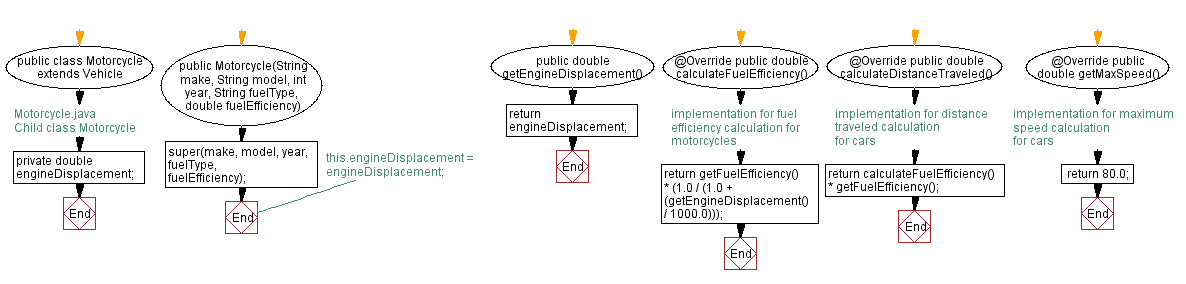Flowchart of Vehicle class Main: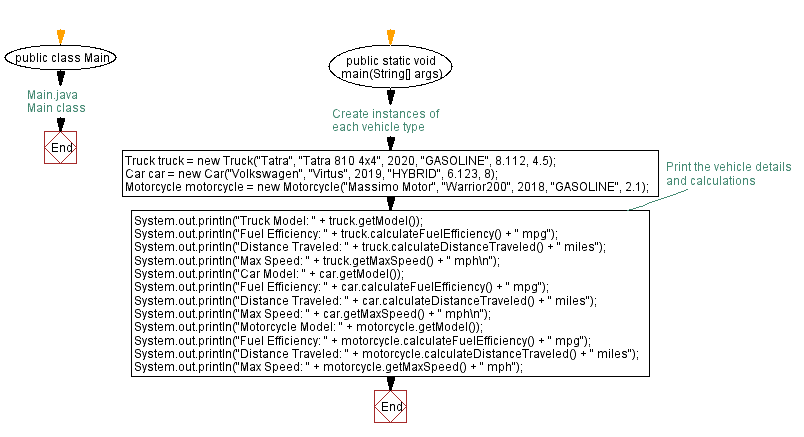Java Code Editor:

What is the difficulty level of this exercise?

﻿

## Java: Tips of the Day

How do I remove repeated elements from ArrayList?

If you don't want duplicates in a Collection, you should consider why you're using a Collection that allows duplicates. The easiest way to remove repeated elements is to add the contents to a Set (which will not allow duplicates) and then add the Set back to the ArrayList:

```Set<String> set = new HashSet<>(yourList);
yourList.clear();